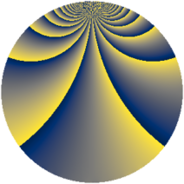# Properties

 Label 441.2.bhLevel $441$ Weight $2$ Character orbit 441.bh Rep. character $\chi_{441}(20,\cdot)$ Character field $\Q(\zeta_{42})$ Dimension $648$ Newform subspaces $1$ Sturm bound $112$ Trace bound $0$

# Learn more

## Defining parameters

 Level: $$N$$ $$=$$ $$441 = 3^{2} \cdot 7^{2}$$ Weight: $$k$$ $$=$$ $$2$$ Character orbit: $$[\chi]$$ $$=$$ 441.bh (of order $$42$$ and degree $$12$$) Character conductor: $$\operatorname{cond}(\chi)$$ $$=$$ $$441$$ Character field: $$\Q(\zeta_{42})$$ Newform subspaces: $$1$$ Sturm bound: $$112$$ Trace bound: $$0$$

## Dimensions

The following table gives the dimensions of various subspaces of $$M_{2}(441, [\chi])$$.

Total New Old
Modular forms 696 696 0
Cusp forms 648 648 0
Eisenstein series 48 48 0

## Trace form

 $$648q - 15q^{2} - 14q^{3} - 57q^{4} - 21q^{5} + 14q^{6} - 5q^{7} - 20q^{9} + O(q^{10})$$ $$648q - 15q^{2} - 14q^{3} - 57q^{4} - 21q^{5} + 14q^{6} - 5q^{7} - 20q^{9} - 28q^{10} - 15q^{11} + 21q^{12} - 7q^{13} - 114q^{14} - 10q^{15} + 39q^{16} - 18q^{18} - 21q^{20} + 10q^{21} + 3q^{22} + 30q^{23} - 14q^{24} + 41q^{25} + 7q^{27} - 20q^{28} + 75q^{29} - 70q^{30} - 39q^{32} - 14q^{33} - 7q^{34} - 128q^{36} - 10q^{37} + 21q^{38} - 36q^{39} - 7q^{40} - 21q^{41} + 104q^{42} + 3q^{43} - 35q^{45} - 72q^{46} - 147q^{47} - 13q^{49} - 18q^{50} + 22q^{51} - 35q^{52} - 14q^{54} - 112q^{55} - 63q^{56} - 16q^{57} + 33q^{58} - 21q^{59} - 90q^{60} - 56q^{61} - 38q^{63} + 52q^{64} + 27q^{65} - 42q^{66} - 26q^{67} - 182q^{69} - 25q^{70} + 24q^{72} - 28q^{73} + 33q^{74} - 14q^{75} + 21q^{76} + 3q^{77} + 90q^{78} - 2q^{79} + 56q^{81} - 28q^{82} - 21q^{83} + 116q^{84} + 5q^{85} - 123q^{86} - 70q^{87} - 41q^{88} - 224q^{90} - 4q^{91} - 225q^{92} + 112q^{93} - 7q^{94} - 12q^{95} - 371q^{96} + 224q^{99} + O(q^{100})$$

## Decomposition of $$S_{2}^{\mathrm{new}}(441, [\chi])$$ into newform subspaces

Label Dim. $$A$$ Field CM Traces $q$-expansion
$$a_2$$ $$a_3$$ $$a_5$$ $$a_7$$
441.2.bh.a $$648$$ $$3.521$$ None $$-15$$ $$-14$$ $$-21$$ $$-5$$